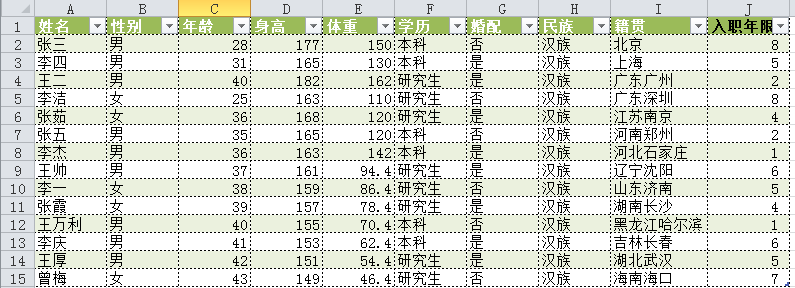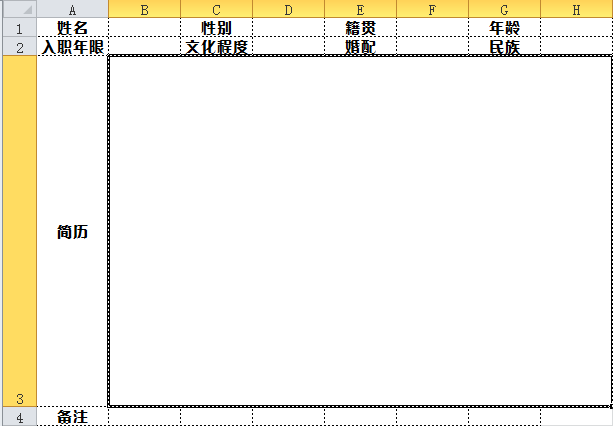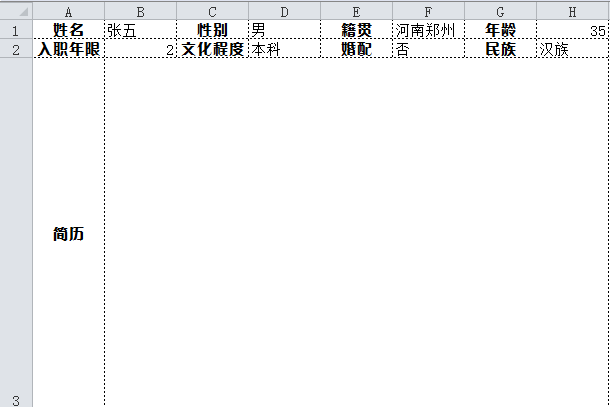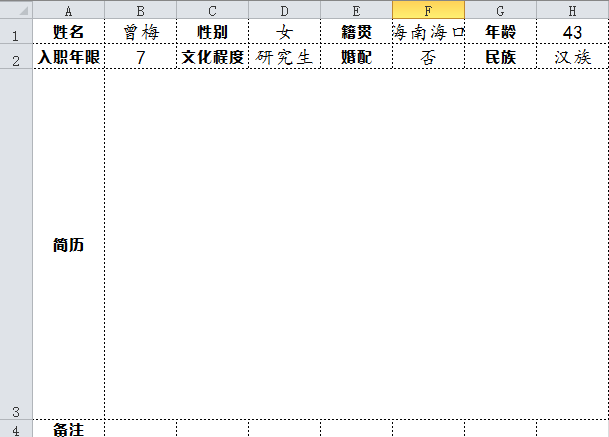# 30 行 Python 代码帮小姐姐填了上百份表格，成功俘获了小姐姐的芳心### 1.用xlwings打开工作簿

import xlwings as xw
workbook=app.books.open(r'D:\数据分析\27.我用python帮小姐姐填了上百份表格\人员信息.xlsx')
sheet=workbook.sheets  #选中第一个表格


### 2.循环每行的数据

info = sheet.used_range
for i in info.raw_value[1:]:
print(i)

('张三', '男', 28.0, 177.0, 150.0, '本科', '否', '汉族', '北京', 8.0)
('李四', '男', 31.0, 165.0, 130.0, '本科', '是', '汉族', '上海', 5.0)
('王二', '男', 40.0, 182.0, 162.0, '研究生', '是', '汉族', '广东广州', 2.0)
('李洁', '女', 25.0, 163.0, 110.0, '研究生', '否', '汉族', '广东深圳', 8.0)
('张茹', '女', 36.0, 168.0, 120.0, '研究生', '是', '汉族', '江苏南京', 4.0)
('张五', '男', 35.0, 165.0, 120.0, '本科', '否', '汉族', '河南郑州', 2.0)
('李杰', '男', 36.0, 163.0, 142.0, '本科', '是', '汉族', '河北石家庄', 1.0)
('王帅', '男', 37.0, 161.0, 94.4, '研究生', '是', '汉族', '辽宁沈阳', 6.0)
('李一', '女', 38.0, 159.0, 86.4, '研究生', '否', '汉族', '山东济南', 5.0)
('张霞', '女', 39.0, 157.0, 78.4, '研究生', '是', '汉族', '湖南长沙', 4.0)
('王万利', '男', 40.0, 155.0, 70.4, '本科', '否', '汉族', '黑龙江哈尔滨', 1.0)
('李庆', '男', 41.0, 153.0, 62.4, '本科', '是', '汉族', '吉林长春', 6.0)
('王厚', '男', 42.0, 151.0, 54.4, '研究生', '是', '汉族', '湖北武汉', 5.0)
('曾梅', '女', 43.0, 149.0, 46.4, '研究生', '否', '汉族', '海南海口', 7.0)

### 3.打开个人信息模板，将信息填入

for i in info.raw_value[1:]:
workbook=app.books.open(r'D:\数据分析\27.我用python帮小姐姐填了上百份表格\个人信息模板.xlsx')
sheet=workbook.sheets
sheet['B1'].value=i
sheet['D1'].value=i
sheet['F1'].value=i
sheet['H1'].value=i
sheet['B2'].value=i
sheet['D2'].value=i
sheet['F2'].value=i
sheet['H2'].value=i### 4.设置单元格格式

list_cell=['B1','D1','F1','H1','B2','D2','F2','H2']#单元格位置
for j in list_cell:
sheet[j].api.Font.Name='楷体'   #设置字体
sheet[j].api.Font.Size=14      #设置字号
#设置文本水平对齐方式为居中
sheet[j].expand('table').api.HorizontalAlignment=xw.constants.HAlign.xlHAlignCenter
#设置文本水平对齐方式为居中
sheet[j].expand('table').api.VerticalAlignment=xw.constants.VAlign.xlVAlignCenter### 5.将表格另存重命名并关闭

workbook.save(r'D:\数据分析\27.我用python帮小姐姐填了上百份表格\{}.xlsx'.format(i))  #以名字命名
workbook.close()
app.quit()
大功告成，看一下效果：



< END >11-0541606-16131
07-12
04-27
05-21